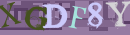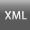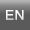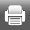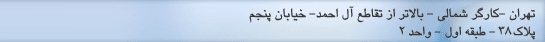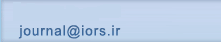[صفحه اصلی ]   [Archive]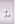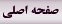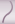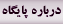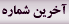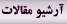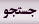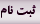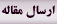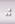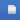جلد 9، شماره 1 - ( 4-1397 )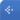جلد 9 شماره 1 صفحات 49-61 برگشت به فهرست نسخه ها
Efficient Algorithm Based on Network Flows for Two-Depot Bus Scheduling Problem
دانشگاه صنعتی بیرجند ، niksirat@birjandut.ac.ir
چکیده:   (330 مشاهده)
In this paper bus scheduling problem under the constraints that the total number of buses needed to perform all trips is known in advance and the energy level of buses is limited, is considered. Each depot has a different time processing cost. The goal of this problem is to find a minimum cost feasible schedule for buses. A mathematical formulation of the problem is developed. When there are two depots, a polynomial time algorithm is developed for the problem and theoretical results about the complexity and correctness of the algorithm is presented. Also, several examples are introduced for illustrating validity of the algorithm.
متن کامل [PDF 455 kb]   (177 دریافت)
نوع مطالعه: پژوهشی | موضوع مقاله: Mathematical Modeling and Applications of OR
دریافت: 1396/1/12 | پذیرش: 1396/12/12 | انتشار: 1397/5/14
ارسال پیام به نویسنده مسئول

ارسال نظر درباره این مقاله
نام کاربری یا پست الکترونیک شما: